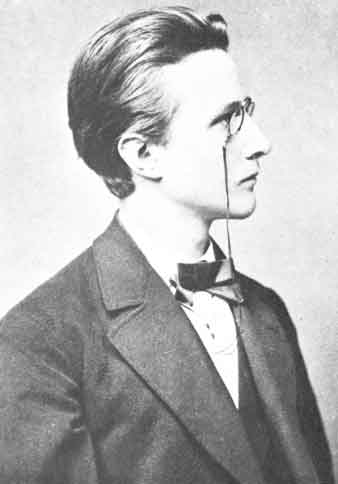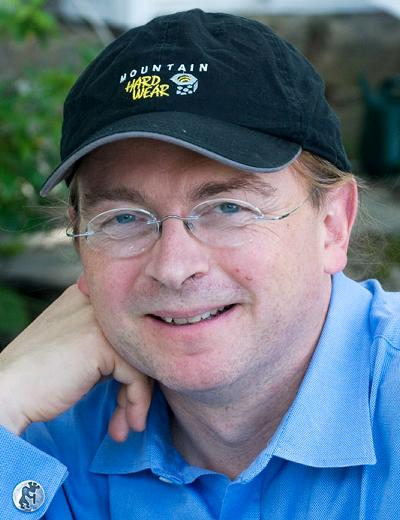## Ψ.1.Natural repetition

### # 1.4.Information resources

#### §1.4.1.Our little universeMax Planck
(1858-1947)

The information capacity of our physical universe was recently estimated by Seth Lloyd at 10^90 quantum bits. Any sequence of bits in a fixed state represents a number – this way the random number the cosmos embodies in an instant is ~ 2^2^299.
There is no particular mathematical reason why there can't be more information around. And indeed, Alan Guth's theories of big bang hyper-inflation provide for an exponential number of pockets of universes of which ours is only `1`.
Whether the size of the larger mathematical physical substrate is unlimited, we do not know.

Fact is, we're stuck with Planck units, quantum particles and astronomical constants, which put a physical lid on the size of the information our universal laptop can process.
Suppose the cosmic processor calculates at a fixed rate of (2×(2×2)!)! Planck impulse moments per Planck time unit of 10^-43.2683 s. This algorithm results in a constant energy in the universe of 10^71.1835 J, which divided by Einstein's square of light speed amounts to 10^54.2298 kg approximately the observed mass in the cosmos.

Most information is packed in dark matter and stellar objects, humanity happens on the fringe of cosmic computation. We wonder, is intelligent life a kind of software corrosion, or are we quality – meant to be – on top of quantity?
If life is important so is ethical behaviour, if the universe is important so is religion. Operating on the edge of randomness and determinacy these bits of life cannot grasp and hold their own raison d'être. On the other hand, this universe – which looks continuous but deep down is discrete – might be a virtual mirror of an earlier Real One, which numerically bugged the Gods called Great. Then what happened to our real selves?
And where are We all?? In a cascade of Transcending importance??? [ write on :-]Seth Lloyd
Bethlehem 2007

According to Seth Lloyd the number of logic operations performed thus far is 10^120 ops. So a digital disk with a perfect copy of the universe up to the present day wastefully puts 10^210 bit configurations in causal order.
This seems to convert ops from time to space, but the cosmic Planck grid counts only 10^60.9 time units up to now, and the laws of nature constantly zip up all quantum events with a physical variant of Jpeg-compression.
Universe, earth, body and mind – all have limited resources. Use them wisely… (read on :-()

Somewhere after stars stopped shining, black holes evaporated and a Big Crunch rolled us all up, the history of the universe must end. We estimate the size of a total copy of cosmic history between 10^100 and 10^300 bits, expressing a random number below the ceiling of 2^2^830 ~ 2^2^2^10.
Left on its own the cosmos never will be able to express a modestly Big random number – for example of size 5^^4 ~ 2^10^2185 ~ 2^2^7257 ~ 2^2^2^13 – even though mathematicians know such numbers exist, in abundance… (this is a proven theory, called the frivolous theorem of arithmetic :-)

Because we can easily express very Big numbers by iteration of functions, Big physical number holes are gaping in-between, where random natural numbers hide which are inexpressible given the resources of our universe.
Big random numbers are physically infinite and cryptographically safe for alien computers (that's a promise ;-)

The way to address random Big individual numbers that hide in physical infinity, is by chance [or prayer ;-]

#### §1.4.2.Odd chances

Just as numbers can get astronomically large, extremely small chances surely exist. This is lovely – you don't know anything that can happen in the real world with 100% certainty. Perhaps your eyes were deceiving you, perhaps someone's memory was incorrect. Small chance? Ask a forensic expert!

Small chances make bad statistics. To win the jackpot in the state lottery 10 times in a row (buying a single ticket for each draw, without using your mafia connections) is less probable than being the first man to have set foot on the moon (when your name isn't Neil Armstrong). Don't you remember? It happened when you were only three – the Russians abducted you, they landed there first (the American moon landings were staged).
Write a scenario, dissect it and multiply the probabilities of each separate event. When you thought of everything and your estimates are right, the resulting product is a lower bound for the total chance, because events tend to correlate and other scenarios are possible too. (This proves the number of connective events in our world is vastly limited.)

To express truly Small chances, we have to refer to processes that can only happen outside of the physical sphere <Guya> of our universe. The proposition that we all live in a yellow submarine which entered a black hole and came out in one piece on the other side, except that your intestines are inside out (of which you'll become aware in about 3 seconds), qualifies with a Small amount of uncertainty as wrong.
Yet it's infinitely more likely than the construction of a spaceship powered by an Infinite Improbability Drive somewhere in the unknown future. But then we're talking infinities, and zero chance.

To think that the number of 9^^^^^^^9 ones shall be reached one day on your computer by click starting the series 1.. (multiple clicks allowed!) and then just letting the program roll out, in our opinion is harder to realize then the aforementioned world inside a submarine.
This belongs in the Guinness Book of Records as the Smallest non-zero chance practically conceived by man (to date in less than 10 characters ;-) And the funny thing is – you can watch your machine make progress. Steadily (even frantically) towards its goal so it seems… Surely the illusion couldn't be Bigger!¡

Nature is random, therefore it's essential to be able to express random numbers and their probability.
We propose these elementary functions of chance.

Let `1? = 0|1 {P(1)= 1/2}` be a flip operation with an outcome of none or one,
both with even chances: ```1?... {#p p↑ω 0*} = p*11**- = p/2```

Define a random number operation `n? = 0|i... {|i#n}`
with an equal probability `P(i)= 1/n1` for each choice, defined as:
```     \$(m,n?)...*n1 {#p↑ω m≤n 0*} = p```

```\$:   \$(a,bi...) = 0 {,bi#k}```  `iff  a≠bj | ... {a≠bj#k}`  `and`
`else \$(a,bi...) = 1 {,bi#k}`  `iff  a=bj & ... {a=bj#k}`